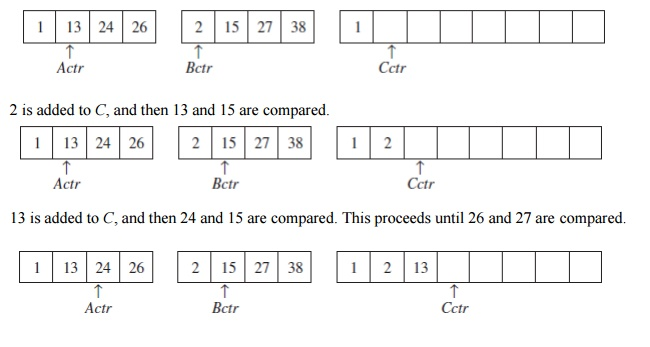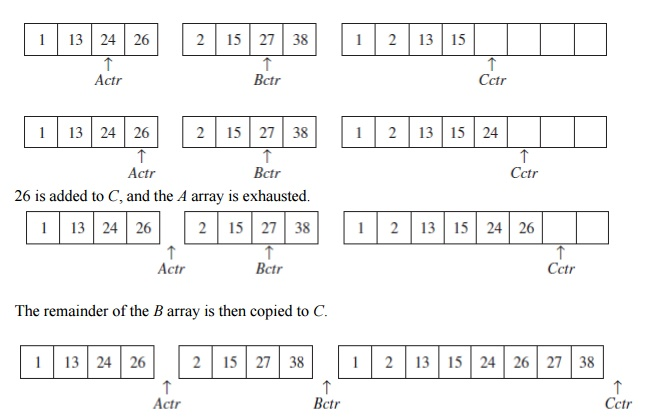# Brief note on Merge Sort

We now turn our attention to mergesort. Mergesort runs in O(N logN) worst-case running time, and the number of comparisons used is nearly optimal. It is a fine example of a recursive algorithm.

Give a brief note on Merge Sort

We now turn our attention to mergesort. Mergesort runs in O(N logN) worst-case running time, and the number of comparisons used is nearly optimal. It is a fine example of a recursive algorithm. The fundamental operation in this algorithm is merging two sorted lists. Because the lists are sorted, this can be done in one pass through the input, if the output is put in a third list. The basic merging algorithm takes two input arrays A and B, an output array C, and three counters, Actr, Bctr, and Cctr, which are initially set to the beginning of their respective arrays. The smaller of A[Actr] and B[Bctr] is copied to the next entry in C, and the appropriate counters are advanced. When either input list is exhausted, the remainder of the other list is copied to C. An example of how the merge routine works is provided for the following input.If the array A contains 1, 13, 24, 26, and B contains 2, 15, 27, 38, then the algorithm proceeds as follows: First, a comparison is done between 1 and 2. 1 is added to C, and then 13 and 2 are compared.The time to merge two sorted lists is clearly linear, because at most N−1 comparisons are made, where N is the total number of elements. To see this, note that every comparison adds an element to C, except the last comparison, which adds at least two. The mergesort algorithm is therefore easy to describe. If N = 1, there is only one element to sort, and the answer is at hand. Otherwise, recursively mergesort the first half and the second half. This gives two sorted halves, which can then be merged together using the merging algorithm described above. For instance, to sort the eight-element array 24, 13, 26, 1, 2, 27, 38, 15, we recursively sort the first four and last four elements, obtaining 1, 13, 24, 26, 2, 15, 27, 38. Then we merge the two halves as above, obtaining the final list 1, 2, 13, 15, 24, 26, 27, 38. This algorithm is a classic divide-and-conquer strategy. The problem is divided into smaller problems and solved recursively. The conquering phase consists of patching together the answers. Divide-and-conquer is a very powerful use of recursion that we will see many times. An implementation of mergesort is provided. The one-parameter mergeSort is just a driver for the four-parameter recursive mergeSort. The merge routine is subtle. If a temporary array is declared locally for each recursive call of merge, then there could be logN temporary arrays active at any point. A close examination shows that since merge is the last line of mergeSort, there only needs to be one

1 /**

2 * Mergesort algorithm (driver).

3 */

4 template <typename Comparable>

5 void mergeSort( vector<Comparable> & a )

6 {

7 vector<Comparable> tmpArray( a.size( ) );

8

9 mergeSort( a, tmpArray, 0, a.size( ) - 1 );

10}

11

12/**

13* Internal method that makes recursive calls.

14* a is an array of Comparable items.

15* tmpArray is an array to place the merged result.

16* left is the left-most index of the subarray.

17* right is the right-most index of the subarray.

18*/

19template <typename Comparable>

20void mergeSort( vector<Comparable> & a,

21vector<Comparable> & tmpArray, int left, int right )

22{

23if( left < right )

24{

25int center = ( left + right ) / 2;

26mergeSort( a, tmpArray, left, center );

27mergeSort( a, tmpArray, center + 1, right );

28merge( a, tmpArray, left, center + 1, right );

29}

30}

Mergesort routines

temporary array active at any point, and that the temporary array can be created in the public mergeSort driver. Further, we can use any part of the temporary array; we will use the same portion as the input array a.

Analysis of Mergesort

Mergesort is a classic example of the techniques used to analyze recursive routines: We have to write a recurrence relation for the running time. We will assume that N is a power of 2 so that we always split into even halves. For N = 1, the time to mergesort is constant, which we will denote by 1. Otherwise, the time to mergesort N numbers is equal to the

1  /**

2  * Internal method that merges two sorted halves of a subarray.

3  * a is an array of Comparable items.

4  * tmpArray is an array to place the merged result.

5  * leftPos is the left-most index of the subarray.

6  * rightPos is the index of the start of the second half.

7  * rightEnd is the right-most index of the subarray.

8  */

9  template <typename Comparable>

10 void merge( vector<Comparable> & a, vector<Comparable> & tmpArray,

11 int leftPos, int rightPos, int rightEnd )

12 {

13 int leftEnd = rightPos - 1;

14 int tmpPos = leftPos;

15 int numElements = rightEnd - leftPos + 1;

16

17 // Main loop

18 while( leftPos <= leftEnd && rightPos <= rightEnd ) 19 if( a[ leftPos ] <= a[ rightPos ] )

20 tmpArray[ tmpPos++ ] = std::move( a[ leftPos++ ] );

21 else

22 tmpArray[ tmpPos++ ] = std::move( a[ rightPos++ ] );

23

24 while( leftPos <= leftEnd ) // Copy rest of first half

25 tmpArray[ tmpPos++ ] = std::move( a[ leftPos++ ] );

26

27 while( rightPos <= rightEnd ) // Copy rest of right half

28 tmpArray[ tmpPos++ ] = std::move( a[ rightPos++ ] );

29

30 // Copy tmpArray back

31 for( int i = 0; i < numElements; ++i, --rightEnd )

32 a[ rightEnd ] = std::move( tmpArray[ rightEnd ] );

33 }

merge routine

time to do two recursive mergesorts of size N/2, plus the time to merge, which is linear. The following equations say this exactly:

T(1) = 1

T(N) = 2T(N/2) + N

This is a standard recurrence relation, which can be solved several ways.We will show two methods. The first idea is to divide the recurrence relation through by N. The reason for doing this will become apparent soon. This yieldsNow add up all the equations. This means that we add all of the terms on the left-hand  side and set the result equal to the sum of all of the terms on the right-hand side. Observe that the term T(N/2)/(N/2) appears on both sides and thus cancels. In fact, virtually all the terms appear on both sides and cancel. This is called telescoping a sum. After everything is added, the final result isNotice that if we did not divide through by N at the start of the solutions, the sum would  not telescope. This is why it was necessary to divide through by N. An alternative method is to substitute the recurrence relation continually on the righthand side. We haveThe choice of which method to use is a matter of taste. The first method tends to produce scrap work that fits better on a standard 81/2 × 11 sheet of paper leading to fewer mathematical errors, but it requires a certain amount of experience to apply. The second method is more of a brute-force approach. Recall that we have assumed N = 2k. The analysis can be refined to handle cases

when N is not a power of 2. The answer turns out to be almost identical (this is usually the case). Although mergesort’s running time is O(N logN), it has the significant problem that merging two

sorted lists uses linear extra memory. The additional work involved in copying to the temporary array and back, throughout the algorithm, slows the sort considerably. This copying can be avoided by judiciously switching the roles of a and tmpArray at alternate levels of the recursion. A variant of mergesort can also be implemented nonrecursively.

The running time of mergesort, when compared with other O(N logN) alternatives, depends heavily on the relative costs of comparing elements and moving elements in the array (and the temporary array). These costs are language dependent. For instance, in Java, when performing a generic sort (using a Comparator), an element comparison can be expensive (because comparisons might not be easily inlined, and thus the overhead of dynamic dispatch could slow things down), but moving elements is cheap (because they are reference assignments, rather than copies of large objects). Mergesort uses the lowest number of comparisons of all the popular sorting algorithms, and thus is a good candidate for general-purpose sorting in Java. In fact, it is the algorithm used in the standard Java library for generic sorting. On the other hand, in classic C++, in a generic sort, copying objects can be expensive if the objects are large, while comparing objects often is relatively cheap because of the ability of the compiler to aggressively perform inline optimization. In this scenario, it might be reasonable to have an algorithm use a few more comparisons, if we can also use significantly fewer data movements

Study Material, Lecturing Notes, Assignment, Reference, Wiki description explanation, brief detail
Object Oriented Programming and Data Structure : Sorting And Searching : Brief note on Merge Sort |